## 4.2. Simulation

### 4.2.1. Mechanics simulation tutorial

This part of the MegaPOV documentation gives a short practical introduction into using the mechanics simulation patch and describes some simple simulations step by step.

#### 4.2.1.1. mass movement

The simplest thing that can be simulated with the mechanics simulation patch is the movement of individual point masses. Their movement under the influence of gravity and environment objects is handled in this part.

We start with a simple scene setup: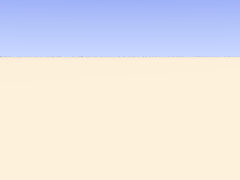To simulate the movement of a single mass we add the following to the global_settings{}:

```mechsim {
gravity <0, 0, -9.81>
method 1

environment {
object plane {  z, 0 }
damping 0.9
friction 0.3
method 2
}

#if (clock_on)
step_count 300
time_step (1/30)/300

topology {
load_file "tut02.dat"
save_file "tut02.dat"
}
#else
step_count 0

topology {
mass { <-2.8, -8.0, 0.9>, <2, 5, 0>, 0.1 density 5000 }
save_file "tut02.dat"
}
#end
}```

The purpose of the individual lines of this block is the following:

gravity <0, 0, -9.81> activates a standard gravity force in negative vertical direction.

method 1 selects simulation method 1 (Euler integration).

The environment {} block contains the definition of the environment. It contains:

• object plane { z, 0 } - the environment object, a plane like seen in the image.

• damping 0.9 - a damping factor decreasing the velocity perpendicular to the surface to 90% during every collision.

• friction 0.3 - a friction factor decreasing the velocity tangential to the surface by 30% during every collision.

• method 2 - activates method 2 collision calculation (impact law based collision).

What follows is an #if conditional. It switches between two different blocks of code depending on whether the scene is rendered as an animation or not. This is useful for initializing the simulation.

The first part contains statements for the animation render:

• step_count 300 - makes MegaPOV calculate 300 simulation steps per frame.

• time_step (1/30)/300 - sets the duration of each step appropriate for a 30 fps animation.

Finding the right step size is probably something most difficult for the beginner. A smaller step size generally leads to more accurate results. Usually the step size has to be below a certain value depending on the other simulation settings to get usable results, but it is not always obvious if some strange result is due to large time steps. High stiffness values in environments, collision and connections usually require smaller time steps. If you experience unexpected results it is often worth trying to decrease the step size and see if problems vanish.

The topology{} block contains just load_file and save_file statements referring to the same file. This makes MegaPOV load the old simulation data at the beginning, calculate the steps for the current frame and save to the same file afterwards.

The second part contains statements for the still render

step_count is set to zero here so no simulation steps are calculated.

The topology{} block contains a singular mass{} section now. It contains:

• <-2.8, -8.0, 0.9> - the starting position of the mass.

• <2, 5, 0> - the initial velocity of the mass.

• 0.1 - the radius of the mass.

• density 5000 - the density of the mass (in kg/m³).

The load_file statement finally saves this mass to a file.

To show the mass in the scene we can use a macro from the mechsim.inc include file:

```#include "mechsim.inc"

MechSim_Show_All_Objects(-1, false, -1, "")```

The meaning of the parameters of this macro is described in Section 3.4.4.2, “MechSim_Show_All_Objects()”.

Now all we need to do is render the scene once as a still to generate the initial data file and afterwards render it as an animation: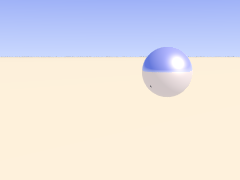#### 4.2.1.2. collisions

In the first part of the tutorial we have handled masses and their movement under the influence of gravity and the constraints of the environment. If several masses exist in the simulation collisions between them have to be taken into account.

For turning collision calculation on the following block is added to the mechsim{} section:

```collision {
1, 0
stiffness 60000
damping 3000
}```

The first two numbers tell MegaPOV which collision to calculate. For details see Section 2.7.3.1.3, “The collision settings”. The numbers used here turn calculation on for all mass-mass collisions.

stiffness (unit kg/s²) and damping (unit kg/s) influence how the masses interact.

Another change made in this tutorial step is changing the environment to function based calculations. This usually leads to more accurate environment collisions, especially with more complex geometries but also tends to be slower and requires smaller integration steps.

Defining the environment as a function is fairy easy with the IsoCSG library. The definition in this case looks like:

```#include "iso_csg.inc"

#declare fn_Env=
IC_Merge5(
IC_Plane(z, 0),
IC_Box(< 1.7,-1.7,-1.0>, < 1.5,1.7,0.5>),
IC_Box(<-1.7,-1.7,-1.0>, <-1.5,1.7,0.5>),
IC_Box(<-1.7, 1.7,-1.0>, <1.7, 1.5,0.5>),
IC_Box(<-1.7,-1.7,-1.0>, <1.7,-1.5,0.5>)
)```

Note that the stiffness, damping and friction parameters in the environment now have the same meaning as in the collision settings because it uses calculation method 1 (force based environment collisions).

This scene also adds new masses during the animation. This is achieved by adding the following code to the topology{} section:

```#if (mod(frame_number, 6)=5)
mass { < 1.3,-1.55,0.8>, <-0.2, 5, 0>, 0.18 density 5000 }
#end```

It means that in every sixth frame a new mass is added at the starting position with the same starting velocity as the first mass.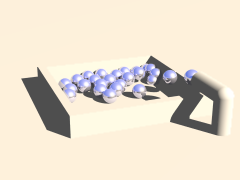#### 4.2.1.3. connections

Apart from masses there is another element that can be added to the topology{} section: connections. Connections can be seen as springs. They connect two point masses and exert a force on them depending on the current distance of the masses compared to the relaxed length of the connection. In addition there is a damping property generating dissipative forces.

A connection{} block is added to the topology{} section like a mass:

`connection { 0, 1 stiffness 50000 damping 2000 }`

It contains:

• 0 - the index of the first mass connected.

• 1 - the index of the second mass connected.

• stiffness 50000 - the stiffness of the connection (unit kg/s²).

• damping 2000 - the damping of the connection (unit kg/s).

if no length is given the current distance of the masses is used.

The mass indices are counted in order of creation starting at zero. The three connected masses from the following scene are created with:

```topology {
mass { <0, 0, 2.4>, <0, 0, 0>, 0.1 density 5000 fixed on }
mass { <1, 0, 2.4>, <0, 0, 0>, 0.1 density 5000 }
mass { <2, 0, 2.4>, <0, 0, 0.6>, 0.15 density 5000 }
connection { 0, 1 stiffness 50000 damping 2000 }
connection { 1, 2 stiffness 50000 damping 2000 }
}```

The fixed on in the first mass definition fixes the position of this mass.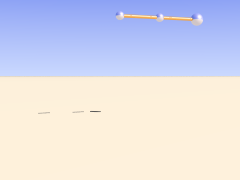#### 4.2.1.4. grids

With plenty of such connections we can form more complex shapes. The mechsim.inc include file (see Section 3.4, “The 'mechsim.inc' include file”) contains several macros forming grids of masses and connections.

Placing the following in the topology{} section generates a box-like grid of connected masses:

```MechSim_Generate_Grid_Std(
<0, 0, 0>, 0.07, 25000, 22000, 2000,
true, <0.4,0.4,0.4>, <3, 12, 3>, Trans1, 3
)```

The meaning of the different parameters is described in Section 3.4.5.4, “MechSim_Generate_Grid_Std()”. Trans1 is a transform moving the whole grid from its predefined position. It has to be declared before:

```#declare Trans1 =
transform {
translate <-0.5, -6*0.4, 1.0>
}```

The resulting animation shows a moving and deforming box geometry.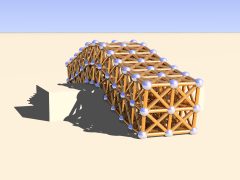The MechSim_Generate_Grid_Std() macro also generates faces for the outside surface of the box. Faces are triangles each connecting three masses. They can be used for collision calculations and for displaying the geometry as a mesh. The latter can be done easily by changing the parameters of the MechSim_Show_All_Objects() macro:

`MechSim_Show_All_Objects(-1, true, -1, "")`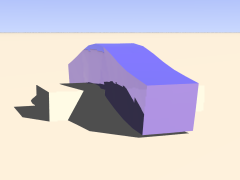#### 4.2.1.5. patches

Apart from 3D grids we of course can also create 2D rectangular patches. Such objects behave like cloth or foil and offer a very interesting field of simulation.

Like 3D grids rectangular patches can be created with macros from the mechsim.inc include file (see Section 3.4, “The 'mechsim.inc' include file”):

```MechSim_Generate_Patch_Std(
<0, 0, 0>, 0.03, 8000, 10000, 0,
true, <0.055, 0.055>, <50, 50>, Trans1, 2
)```

This creates a 50x50 masses patch, again movable with a transform. Description of the parameters can be found in Section 3.4.5.7, “MechSim_Generate_Patch_Std()”.

The following sample scene also uses a different simulation method than the previous scenes. It is called gradient descent method. It does not integrate the equations of movement like the other methods but moves the masses directly according to the forces influencing them. Inertia does not have effect with these method, therefore oscillations can't occur. Still the step size has to be chosen small enough for accurate results. The movement won't look very realistic in an animation but the final result can be used for a still render. Another disadvantage is the lack of friction, therefore the patch slides off the sphere in the end.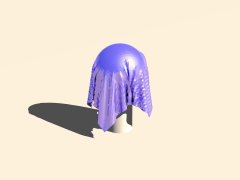There is also a specialized macro for generating a mesh from a patch topology including normal vectors and uv coordinates. Rendering the previous topology with this macro results in the following picture: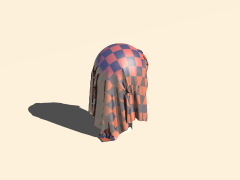There are a lot more aspects of the mechanics simulation patch that are not handled in this tutorial yet. Reading the reference (see Section 2.7.3, “Mechanics simulation patch”) and mechsim.inc include file documentation (see Section 3.4, “The 'mechsim.inc' include file”) should help learning about those things. The sample scenes show several examples what the simulation system can be used for but there are a lot more possibilities.